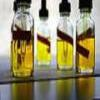#### You may also like### Mathematical Issues for Chemists

A brief outline of the mathematical issues faced by chemistry students.### Reaction Rates

Explore the possibilities for reaction rates versus concentrations with this non-linear differential equation### Catalyse That!

Can you work out how to produce the right amount of chemical in a temperature-dependent reaction?

# Extreme Dissociation

##### Age 16 to 18Challenge Level

The pH of a solution is defined using logarithms as

$$pH = -\log_{10}[H^+],$$
where $[H^+]$ is the concentration of $H^+$ ions in mol/l of the solution.

Imagine that this equation works for any concentration of $H^+$, and imagine that scientists have created conditions in a 100ml sample of pure water in which only one molecule of water has dissociated into $H^+$ and $OH^{-}$ (such conditions are, or course, science fiction!)

What would the pH of this fluid be?

Next imagine that conditions arise in which every single molecule of water dissociates.

What would the pH be now? What assumptions do you make in the calculation?

Under real-world situations, what volume of water would we expect to contain exactly one $H^+$ ion?

###### Send feedback on this problem

NOTES AND BACKGROUND
This problem uses the concept of dissociation in which molecules of water $H_2O$ dissociate into $H^+$ and $OH^{-}$ ions. In reality, the pH formula given here fails to hold for weak acids or bases because not all of the water in the solution splits into $H^+$ and $OH^-$ ions. Only some of the water will dissociate; the dissociation constant tells you how many molecules split in this way.

Whilst the pH scale is usually quoted as running from 1-14, very strong acids can have zero or even negative pH values, running as low as -20 for superacids .